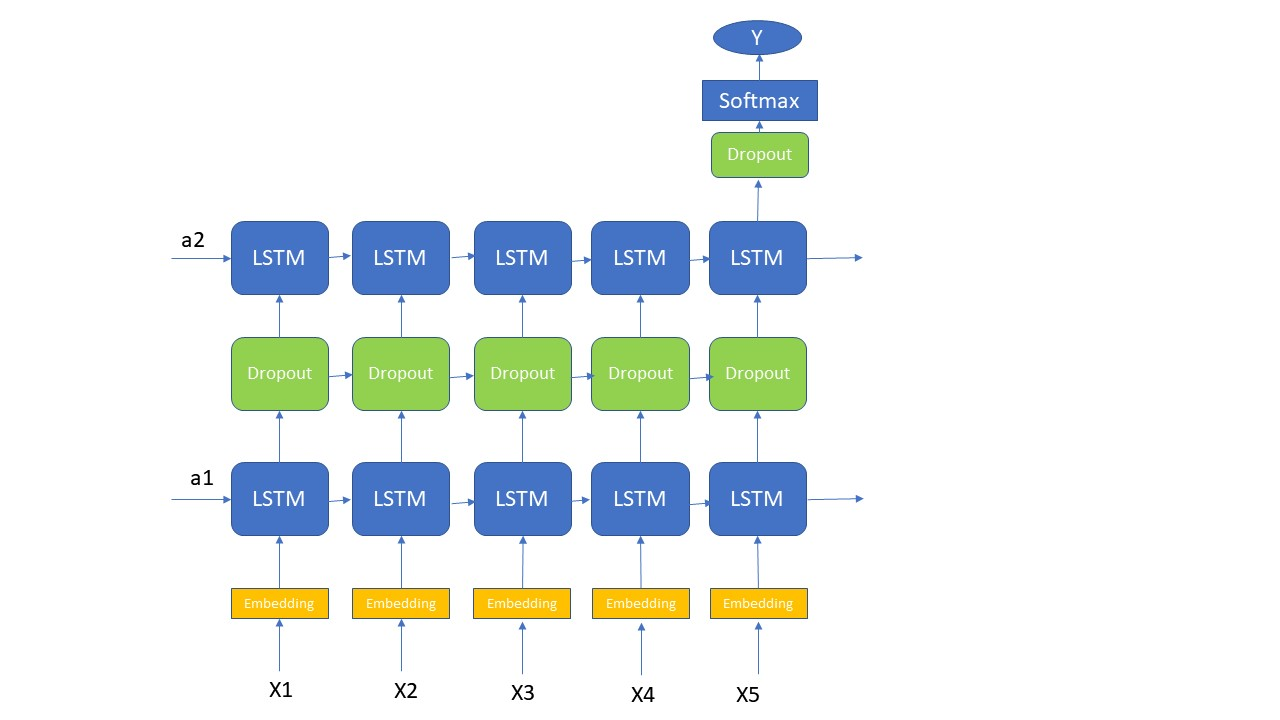# 机器学习 - LSTM应用之情感分析

2019/04/10 10:10

1. 概述

2. 结构设计3.神经网络结构的代码实现

def Sentiment_Model(input_shape):
"""
Function creating the Emojify-v2 model's graph.

Arguments:
input_shape -- shape of the input, usually (max_len,)

Returns:
model -- a model instance in Keras
"""

# Define sentence_indices as the input of the graph.
# It should be of shape input_shape and dtype 'int32' (as it contains indices, which are integers).
sentence_sequences = Input(input_shape, dtype='int32')
# Propagate sentence_indices through your embedding layer
# (See additional hints in the instructions).
embeddings = Keras.Embedding(sentence_sequences)

# Propagate the embeddings through an LSTM layer with 128-dimensional hidden state
# The returned output should be a batch of sequences.
X = LSTM(128, return_sequences = True)(embeddings)
# Add dropout with a probability of 0.5
X = Dropout(0.5)(X)
# Propagate X trough another LSTM layer with 128-dimensional hidden state
# The returned output should be a single hidden state, not a batch of sequences.
X = LSTM(128, return_sequences=False)(X)
# Add dropout with a probability of 0.5
X = Dropout(0.5)(X)
# Propagate X through a Dense layer with 5 units
X = Dense(5)(X)
# Add a softmax activation
X = Activation('softmax')(X)

# Create Model instance which converts sentence_indices into X.
model = Model(inputs=sentence_sequences, outputs=X)

return model

4. 总结

这里主要讲述了一个Many-to-One结构的LSTM的一般应用，这里主要是用一个情感分析的例子来演示，但是它的应用非常广泛的。这里的重点是对LSTM 的Many-to-One结构的理解，其次是对于多层LSTM layer的应用和理解。至于用TensorFlow来实现这个神经网络的部分，主要是对LSTM 中的一些参数的设置要理解就行了，其他的都很简单。### 作者的其它热门文章

0
0 收藏

0 评论
0 收藏
0